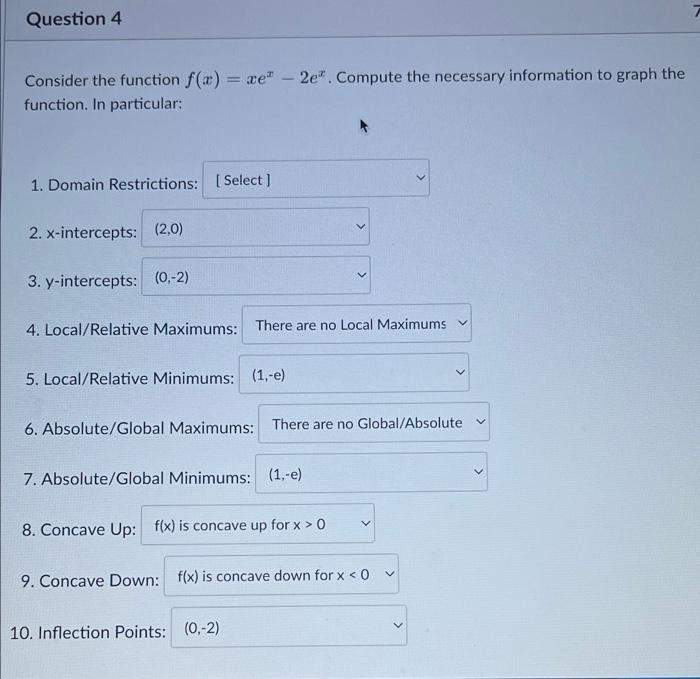Home / Expert Answers / Calculus / question-4-consider-the-function-f-x-xe-2e-compute-the-necessary-information-to-graph-the-funct-pa765

# (Solved): Question 4 Consider the function f(x) = xe* 2e. Compute the necessary information to graph the funct ...

Question 4 Consider the function f(x) = xe* 2e. Compute the necessary information to graph the function. In particular: 1. Domain Restrictions: [Select] 2. x-intercepts: (2,0) 3. y-intercepts: (0,-2) - 4. Local/Relative Maximums: There are no Local Maximums ✓ 5. Local/Relative Minimums: (1,-e) 6. Absolute/Global Maximums: There are no Global/Absolute 7. Absolute/Global Minimums: (1,-e) > 8. Concave Up: f(x) is concave up for x > 0 10. Inflection Points: (0,-2) 9. Concave Down: f(x) is concave down for x < 0 >Consider the function . Compute the necessary information to graph the function. In particular:

We have an Answer from Expert